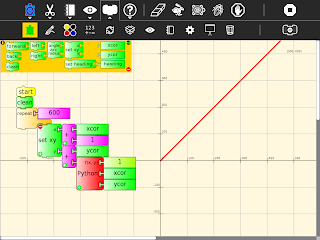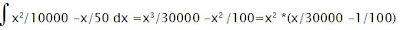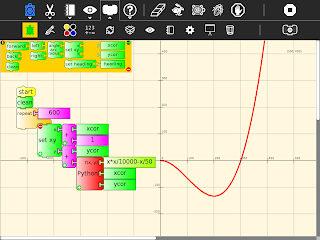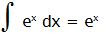## Sunday, May 08, 2011

### Turtle calculus

Its easy to plug in various functions as a graph's gradient in Turtle Art. The resulting graph is the integral of the function used as the gradient. In the below case, the gradient is 1 and its integral is a straight line, f(x)=xPlug in a linear gradient, -2+x/100, and make a parabola with zero crossings at x=0 and x=400A quadratic gradient generates a cubic with zero crossings at x=0 and x=300The integral of sin is -cos(by the chain rule)making dy/dx =y gives an exponential curvebut a nonzero value at x=0 is necessary, in this case forward 1 means f(0)=1
starting at 0, f(0)=0 has a solution of a straight line f(x)=0Challenges
Graph for negative x
Graph the exponential for f(0)=-1 explain
The exponential above is for dy/dx = y/100 what exactly is the formula for the graph?
Find more interesting integrals
Graph the derivative of a function
Iteratively solve d2y/dx2 =-y
Explain the offset in the graph of -cos
A step size of 1 is used, what error does the step size introduce?

Labels: , , , , ,